# How to Generate Random date values in Excel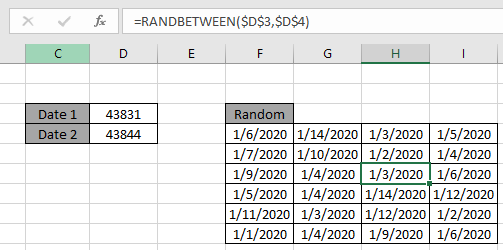In this article, we will learn to generate random date in Excel. You can use the mentioned formula as a date randomizer too.

Scenario:

Let's say we need random dates between two given dates for any given reason. So how do we do that?

How to solve the problem.

The dates are basically serial numbers  in Excel. So we just need a formula where we can input dates as numbers and get the return result in date format. Here the solution to the problem depends on the condition. Possible conditions can be as follows.

1. Date values between any two given dates.
2. Date value within any given year.
3. Date values from a small list of date values.
4. Date values from a long list of data values.

So we will get to all the above problems but for that first we need to understand a little about the Excel RANDBETWEEN function.

The first problem is where the two dates are given in as direct argument to the function.
Use the formula:

= RANDBETWEEN ( date1, date2 )

date1 : the bigger date as top

date2 : the smaller date as bottom

Example: Create a List of Random Dates Between Two Defined Dates.

Here we have given two different dates in cell D3 & D4.

Now we need a bunch of random dates between the two given dates.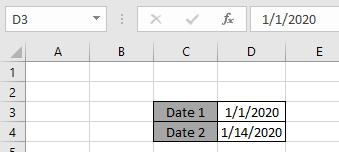Now we will be using the formula to generate the date values.

Use the formula:

= RANDBETWEEN ( D3 , D4 )

Explanation:

• The dates in serial number can be determined as shown below.• So the formula just returns a number between the these given numbers which then can be converted to the date format.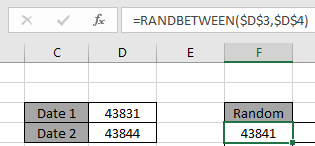• The returned number can be converted to date value via changing the format of the cell to a short date. The dollar sign ( \$ ) freezes the cell reference.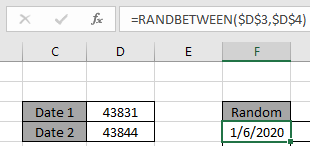• Now drag down and right to generate as many number of date value as you want.As you can see the formula returns the date value between the two given dates.

Now the second formula for another problem is getting random date within an year. So assume we need date values from the year 2019. We will use the DATE function with the RANDBETWEEN function.

Use the formula:

= RANDBETWEEN ( DATE (2019,1,1) , DATE (2019,12,31))

Explanation:

• DATE function generates date value which can be used as an argument to the RANDBETWEEN function.
• DATE (2019,1,1) returns the 1/1/2019 the first date of the year & DATE(2019,12,31) returns the 12/31/2019 the last date of the year.
• The RANDBETWEEN function returns numbers whcih are in fact the date value when converted to date format.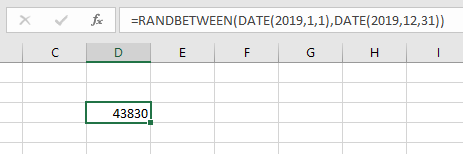After changing the cell to Short date format.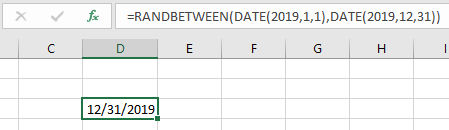Now drag down and right to generate as many number of date value as you want.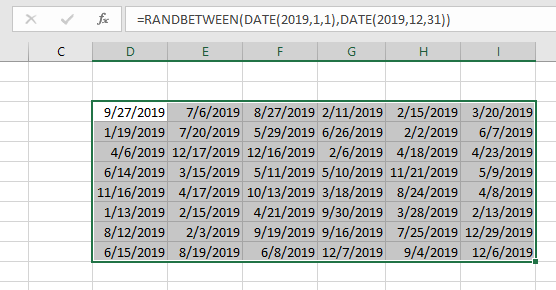As you can see the formula works fine and returns the random date values within year 2019.

Now we switch to a third kind of formula which is generating random date values form the given list of date values. For this we can use either of the two formulas. First is using it when the given list of date value is small.

Use the formula:

= CHOOSE ( RANDBETWEEN ( 1 , 7 ), A1 , A2 , A3 , A4 , A5 , A6 , A7 )

Explanation:

1. CHOOSE function returns the value from the list of values from the index returned by the randbetween function.
2. The index will be generated by the randbetween FUNCTION
3. The CHOOSE function just returns the value as per index value.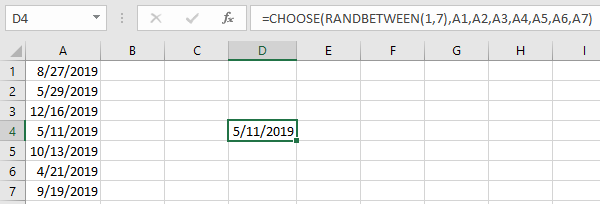Now drag down or right to get as many number of date values. But first freeze the cell reference.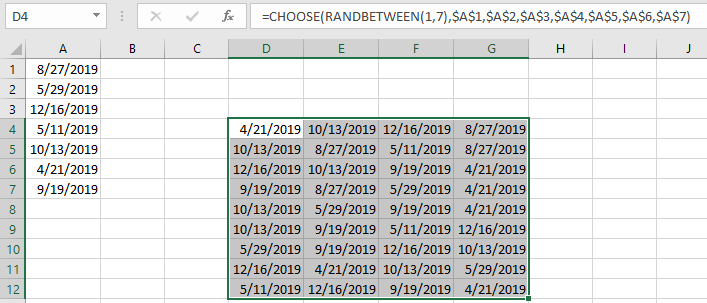As you can see the formula returns all the date values from the given list of date values.

Now for the fourth kind which is long list of date values

For this, we will be needing the use the following functions:

Now we will make a formula out of these functions. Here we will give the range to the INDEX function from where it can generate different values from the list.

Use the formula:

=INDEX ( date , RANDBETWEEN ( 1 , ROWS ( date ) ))

Explanation:

• ROWS function will return the length of the list or data.
• RANDBETWEEN will generate a number between 1 to number of values in the list.
• INDEX function returns the value from data depending on the number generated by the RANDBETWEEN function.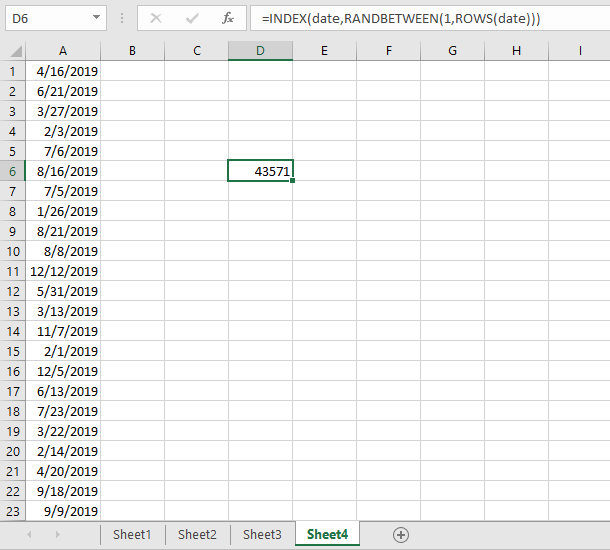Now drag down or right to get as many number of date values. For this you don't need to freeze any reference as the named range is already a fixed reference.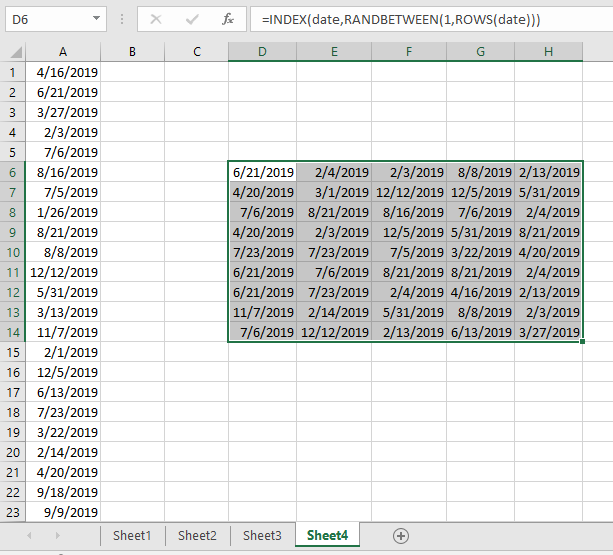As you can see the formula returns all the date values from the given list of date values.

As you can see in the above snapshot the outcomes of the formula.

Notes :

1. The RANDBETWEEN function returns an error, if the first number argument ( bottom ) is larger than the second number argument ( top ).
2. The date value must be given as cell reference or using the DATE function or else the function return
3. The RANDBETWEEN function returns error, if the argument to the function is non - numeric.
4. The CHOOSE function returns #VALUE! Error if the value argument to the function is given as array.
5. The CHOOSE function returns error if the returned number by the RANDBETWEEN function exceeds the number of values.

Hope this article about how to generate random date values in Excel is explanatory. Find more articles on TEXT functions here. Please share your query below in the comment box. We will assist you.

Related Articles

Generate Random Phone Numbers

Get Random number From Fixed Options

Get Random numbers between two numbers

Excel Random Selection: How to Get Random Sample From a Dataset

How to use the CHOOSE Function in Excel

How to use the RANDBETWEEN Function in Excel

Popular Articles

50 Excel Shortcut to Increase Your Productivity

Edit a dropdown list

Absolute reference in Excel

If with conditional formatting

If with wildcards

Vlookup by date

Convert Inches To Feet and Inches in Excel 2016

Join first and last name in excel

Count cells which match either A or B

Terms and Conditions of use

The applications/code on this site are distributed as is and without warranties or liability. In no event shall the owner of the copyrights, or the authors of the applications/code be liable for any loss of profit, any problems or any damage resulting from the use or evaluation of the applications/code.